# Consumer’s Budget- Budget set and Budget Line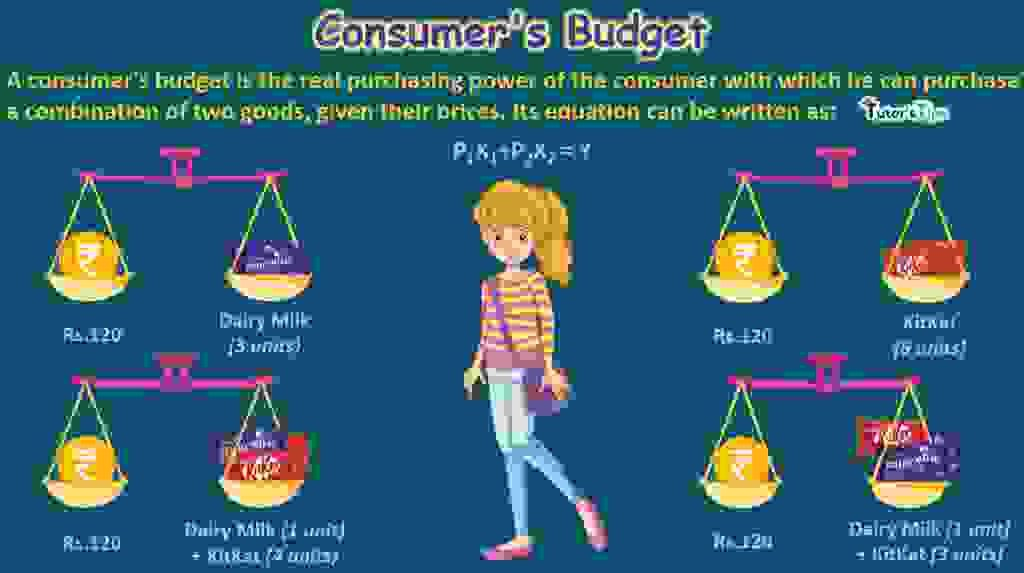Consumer Budget including budget set and budget line refers to the actual buying capacity of buyer with which he can purchase a combination of commodities at given prices. It is represented by the budget set and budget line. The indifference curve and consumer budget are used to determine the consumer’s equilibrium.

The Content covered in this article:

## What is Consumer Budget?

A consumer’s budget is the real purchasing power of the consumer with which he can purchase a combination of two goods, given their prices. It shows the combinations of goods and services which a consumer can buy with his limited income.

For instance, suppose a customer has a fixed income portion to spend on two commodities. The prices of both commodities are fixed in the market. He will choose the best among the combination of two goods giving him maximum satisfaction. Given his fixed income and cost of commodities in the market, the customer can manage to buy the combination of goods which yields more utility to him as compared to the price paid.

Consumer Budget includes:

1. Budget Set
2. Price or Budget Line

Subscribe our Youtube Channel

### What is Budget Set :

It refers to attainable combinations of a set of goods, given prices of goods and income of the consumer. The budget set equation can be written as:

P1X1+P2X2 ≤ Y

Here,

P1 refers to the price of Good-1

Xrefers to the quantity of Good-1

Prefers to the price of Good-2

X2 refers to the quantity of Good-2

Y refers to total expenditure or total budget.

Budget set is also known as a budget constraint as it shows the limit up to which a consumer can buy a set of two goods with given income.

#### Representation:

Suppose a customer has a fixed income of Rs.320 to be spent on wheat and rice. Price of rice is Rs.80 per unit and that of wheat is Rs.20 per unit. With his given income and prices, the different combinations a consumer can get, are:

 Income (in thousands) Rice (@Rs.80per unit) Wheat(@Rs.20per unit) 320 0 16 320 1 12 320 2 8 320 3 4 320 4 0

The above table shows that if a consumer wants to buy only wheat, he can get a maximum of 16 units of wheat with his income of Rs.320. On the other hand, if he wants to buy only rice, he can get a maximum of 4 units of rice with the entire income of Rs.320. Within these two limits, the possible combinations are 1 unit of rice+12 units of wheat, 2 units of rice+ 8 units of wheat and 3 units of rice and 4 units of wheat.

### What is the Budget line:

Budget Line is a line showing different possible combinations of Commodity-1 and Commodity -2 which a consumer can buy within his budget and given market prices of commodities. It is also known as price line, consumption possibility line and line of attainable combinations.

The basic components of this line are:

1)Consumer’s Purchasing power (income)

2)Price or market value of two goods.

Its equation can be written as:

P1X1+P2X2 = Y

Here,

P1 refers to the price of Good-1

Xrefers to the quantity of Good-1

Prefers to the price of Good-2

X2 refers to the quantity of Good-2

Y refers to total expenditure or total budget.

#### Definitions:

In the words of Ferguson,

“The price line shows the combinations of goods that can be purchased if the entire money income is spent.”

According to Hibdon,

” The price line shows all the different combinations of the two commodities that a consumer can purchase given his money income and the price of two commodities.”

#### Graphical Representation:

The budget or price line can be represented as :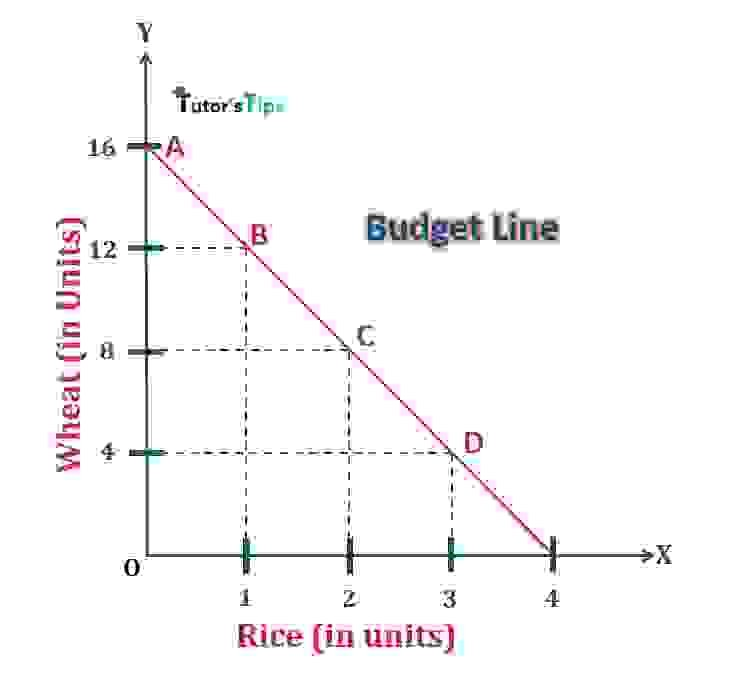Budget Line

In fig, X-axis shows the quantity of rice and Y-axis shows the quantity of wheat. The price line denotes different combinations of rice and wheat. If there is any point beyond this line, the consumer is unable to buy any combination of these good with his limited income. It is presumed that the consumer spends his entire income on the consumption of these two goods only. Thus, the budget or price line is the limit line of the consumer.

#### Properties of Budget Line :

If the prices of the two commodities are fixed in the market, then the price line possesses the following properties:

##### 1. The budget line is a straight line:

Since this line is derived from a linear equation P1X1+P2X2 = Y, it is denoted by a straight line. The intercepts on the axes show the maximum quantity of a commodity one can purchase when no other commodity is purchased.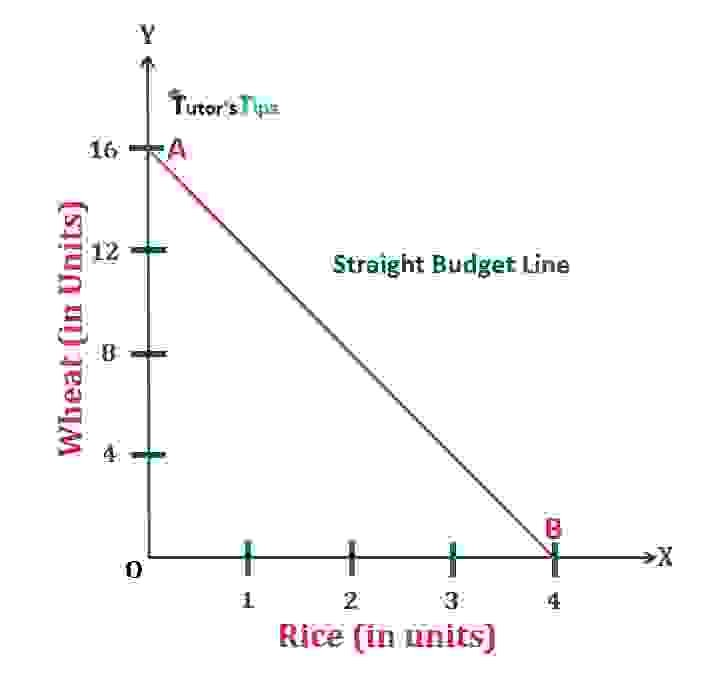1.Straight Budget Line- Properties of Budget line

In Fig, X-axis shows the quantity of rice and Y-axis shows the quantity of wheat. AB curve represents the budget or price line. At point A, the purchase of rice by the consumer is nil, whereas, at point B, the consumer prefers to purchase only rice.

##### 2. It slopes downward as it has a negative slope:

The budget/price line slopes from left to right. The slope of this line can be expressed as Px/Py.  Thus, it slopes downward as an increase in consumption of one commodity declines the consumption of the other one.

##### 3. Parallel Budget Lines:

As long as the price ratio of commodities is the same, the slope of the budget or price lines is also the same. The same proportionate change in prices of commodities results in parallel budget lines.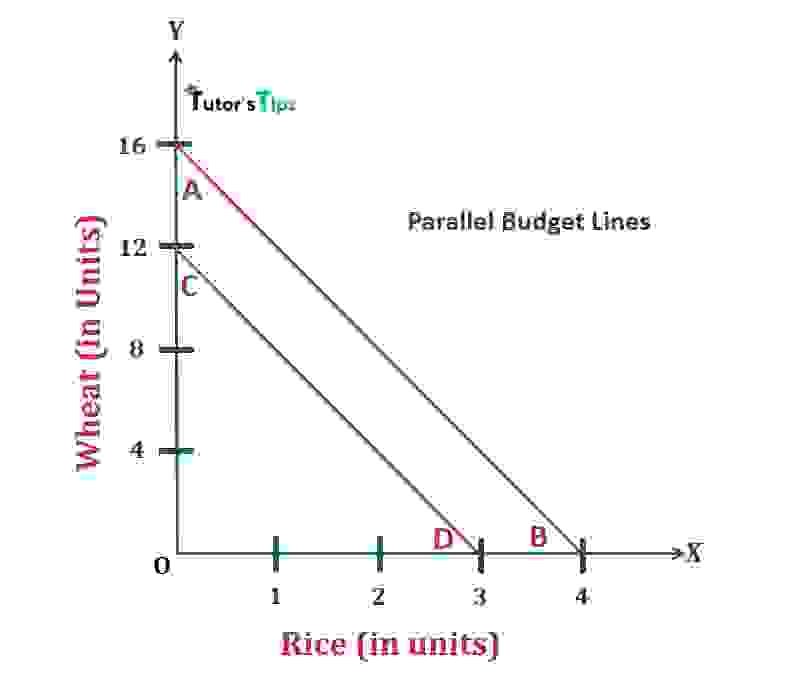3.Parallel budget lines-Properties of Budget Line

In fig, AB and CD are budget lines. It shows the impact of the same proportionate change in the prices in both commodities. If the prices of both commodities change proportionately, the budget lines wouldn’t be parallel. However, if the prices of commodities and income of consumer change in the same proportion, the budget would be the same.

##### 4.Any point beyond the budget line shows non-attainable or non-feasible combinations:

It denotes a line which divides the region into two parts i.e. feasible and non-feasible portion. The right-angled triangle formed by the price line with the axes is the feasible portion where the consumer can afford the combinations of two goods within his income at given prices. On the other hand, the portion beyond the line represents non-feasible combinations of two goods which one cant afford.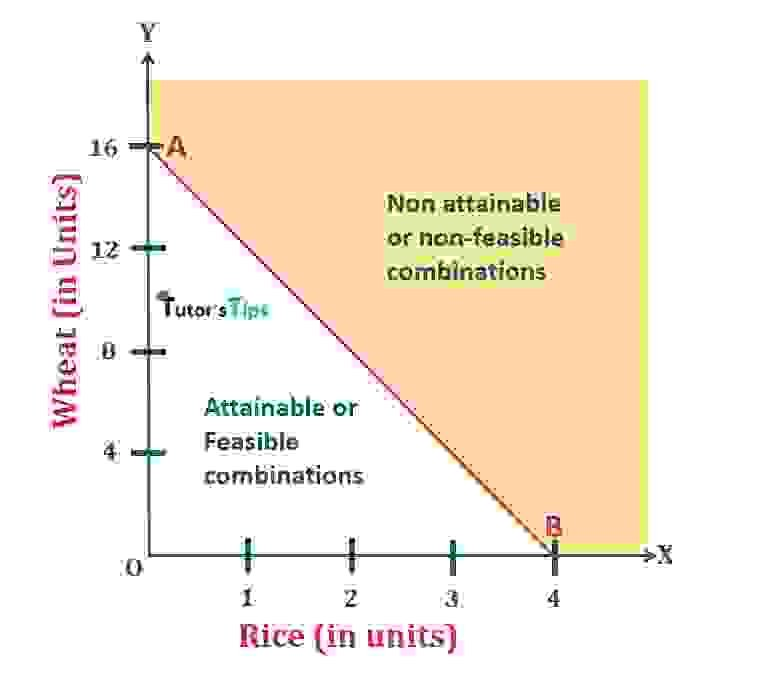4.Non-feasible combinations-Properties of Budget Line

In fig, AB is the price line. The shaded area beyond this line represents the non-attainable or non-feasible combinations of two commodities to consumers. However, at any point below this line, the consumer can afford the combinations with his income, given the prices.

##### 5. When the income of consumer increases, the budget line shifts forward without changing its slope:

If the prices of both commodities remain the same, then an increase in the income of consumer shifts the budget or price line to left. In other words, the price line shifts forwards when the income of the consumer increases with a constant price.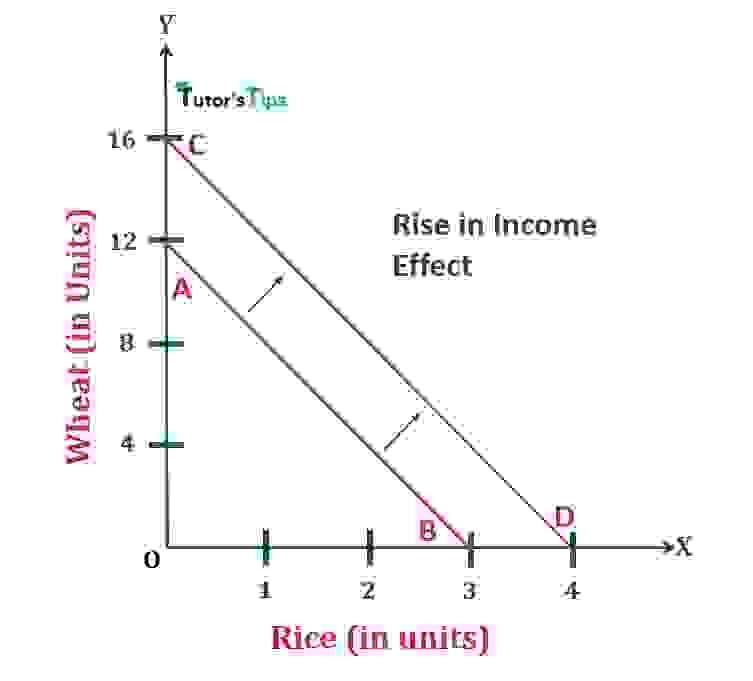5.The forward shift- Properties of Budget Line

In Fig, When the income of a consumer is Rs240, he could buy the combination as represented by the budget/price line AB. When his income increases to Rs.320, keeping the prices same, the consumer is able to buy more of both commodities. Now, He can buy a maximum of 4 units of rice instead of 3 units and a maximum of 16 units of wheat instead of 12 units. With the increase in income, the Budget line shifts from AB to CD.

##### 6. The budget line shifts backwards when the income of consumer decreases, without changing its slope:

If the prices of both commodities remain the same, then the decrease in the income of consumer shifts the budget or price line to the right. In other words, the budget line shifts backwards when the income of the consumer decreases at a constant price.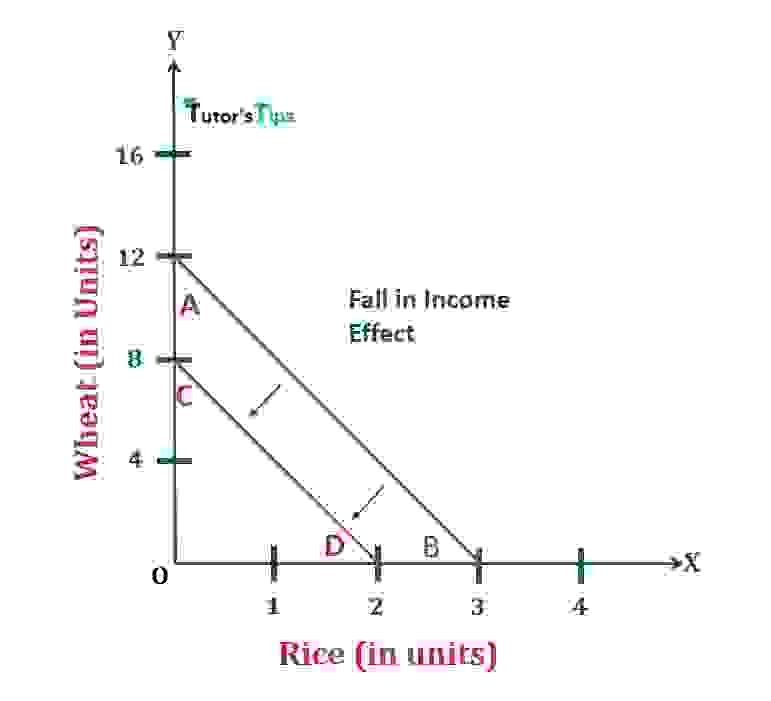6.The backward shift-Properties of Budget Line

In Fig, When the income of a consumer is Rs240, he could buy the combination as represented by the budget/price line AB. When his income decreases to Rs.160, keeping the prices same, the consumer is able to buy less of both commodities. Now, He can buy a maximum of 2 units of rice instead of 3 units and a maximum of 8 units of wheat instead of 12 units. With the decrease in income, the Budget line shifts from AB to CD.

##### 7. Fall in Price of one Commodity:

If the income and price of one commodity remain the same, but the price of another commodity falls, the budget or price line shifts to the right. The curve touching the axis of that commodity of which the price has fallen shifts forward from its original place.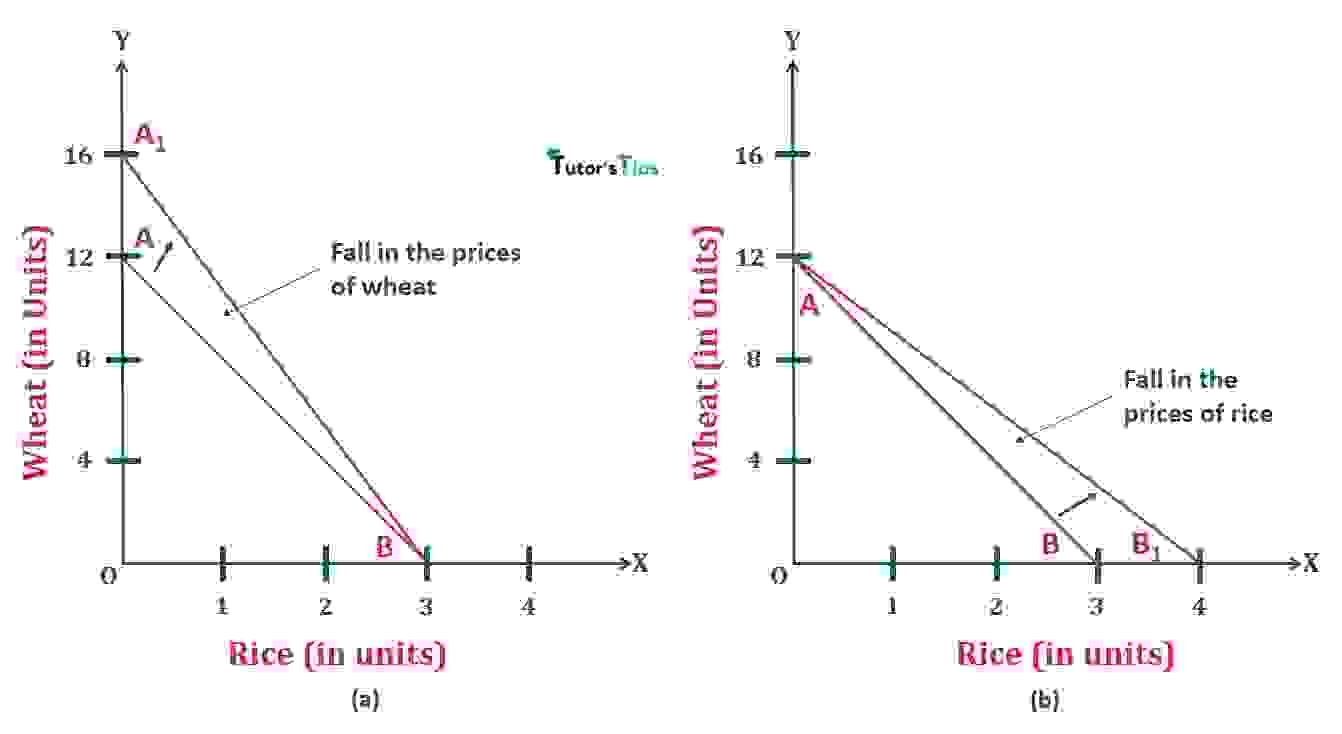7.Fall in the price of one commodity-Properties of Budget Line

In fig, (a)  and (b) shows the effect of fall in prices of wheat and rice respectively. In (a), AB is the initial price line. When the price of wheat falls while the income of consumer and prices of rice constant, then the slope of the budget/price line shift from AB to A1B. In this new situation, the consumer is able to buy more units of wheat. Similarly in (b), when the price of rice falls, keeping the income and price of wheat constant, then the slope of budget line shift from AB to AB1. In this new situation, the consumer is able to buy more units of rice.

##### 8. A rise in Price of one Commodity:

If the income and price of one commodity remain the same, but the price of another commodity rises, the budget/price line shifts to the left. The curve touching the axis of that commodity of which the price has risen shifts backwards from its original place.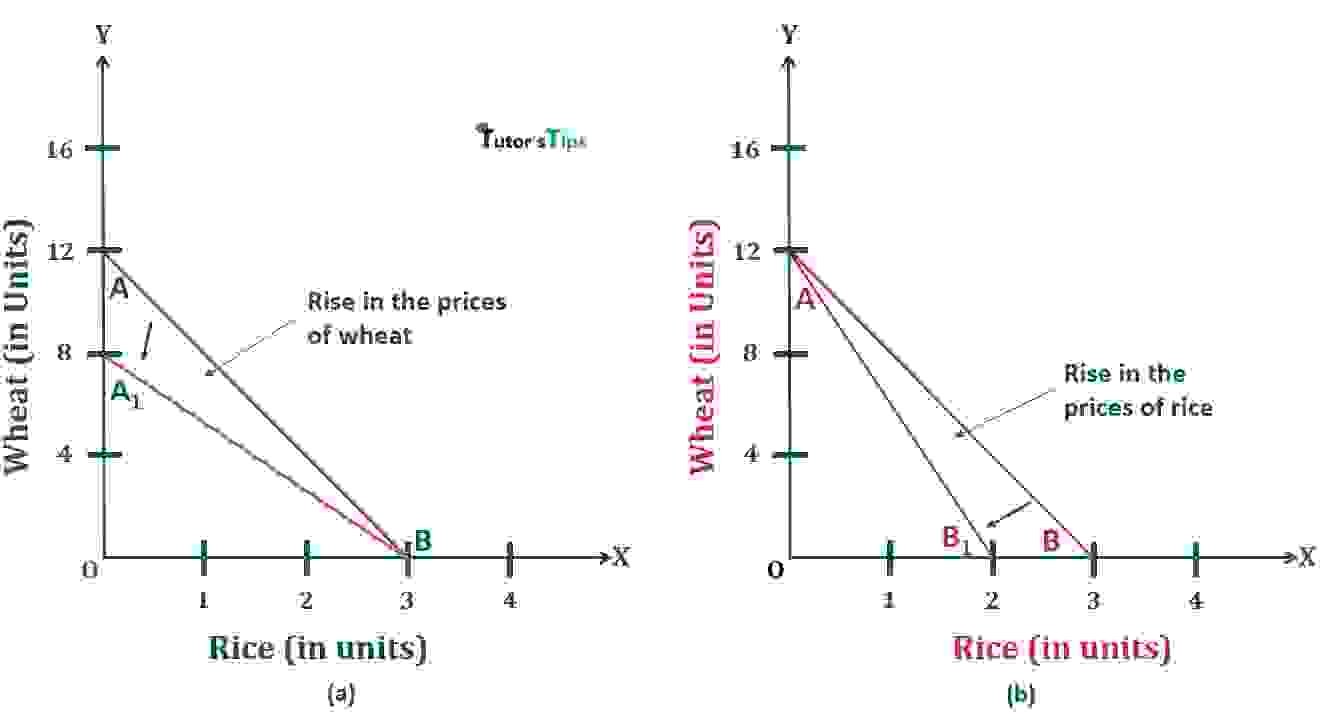8.The rise in Price of one commodity-Properties of Budget Line

In fig, (a)  and (b) shows the effect of the rise in prices of wheat and rice respectively. In (a), AB is the initial budget/price line. When the price of wheat increases while the income of consumer and prices of rice constant, then the slope of this line shift from AB to A1B. In this new situation, the consumer is able to buy fewer units of wheat. Similarly in (b), when the price of rice rises, keeping the income and price of wheat constant, then the slope of budget line shift from AB to AB1. In this new situation, the consumer is able to buy fewer units of rice.

Thanks Please share with your friends

Comment if you have any question.

References:

Introductory Microeconomics – Class 11 – CBSE (2020-21)